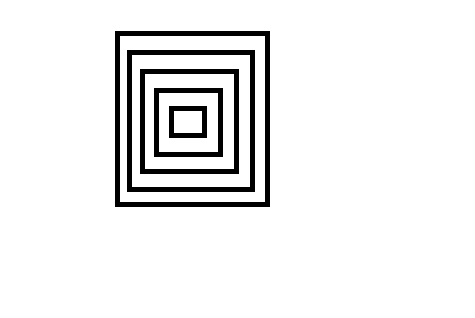Question 62

# The adjoining figure shows a set of concentric squares. If the diagonal of the innermost square is 2 units, and if the distance between the corresponding corners of any two successive squares is 1 unit, find the difference between the areas of the eighth and the seventh squares, counting from the innermost square.Solution

Diagonal of 8th square will be = 16
Side of 8th square = $$\frac{16}{\sqrt2}$$
Diagonal of 7th square will be = 14
Side of 7th square = $$\frac{14}{\sqrt2}$$

Difference in areas = $$(\frac{16}{\sqrt2})^2 - (\frac{14}{\sqrt2})^2$$ = 30